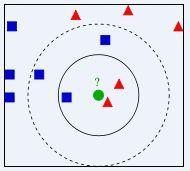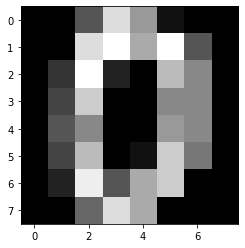# 近邻算法介绍### 原理

KNN是通过测量不同特征值之间的距离进行分类。思路是：如果一个样本在特征空间中的 K 个最相似(即特征空间中最邻近)的样本中的大多数属于某一个类别，则该样本也属于这个类别。其中 K 通常是不大于 20 的整数。KNN算法中，所选择的邻居都是已经正确分类的对象。该方法在定类决策上只依据最邻近的一个或者几个样本的类别来决定待分样本所属的类别。

﻿1378215-20180805232806939-472376897.png

﻿

KNN 算法计算过程

﻿

1. 计算待分类物体与其他物体之间的距离；

2. 统计距离最近的 K 个邻居，按照距离递增关系进行排序；

3. 选取距离最小的 K 个点；

4. 确定前 K 个点所在类别的出现频率；

5. 返回前K个点中出现频率最高的类别作为测试数据的预测分类

﻿

K 值的选择

﻿

﻿

KNN 算法适用场景

﻿

KNN 算法可以用作分类，也可以用作回归，数据量不大场景下，也可以作为推荐算法使用。

﻿

### 案例

﻿

from sklearn.model_selection import train_test_splitfrom sklearn.datasets import load_digitsfrom sklearn.neighbors import KNeighborsClassifierfrom sklearn.tree import DecisionTreeClassifierimport matplotlib.pyplot as plt# 加载数据digits = load_digits()data = digits.data# 数据探索print(data.shape)# 查看第一幅图像print(digits.images)# 第一幅图像代表的数字含义print(digits.target)# 将第一幅图像显示出来plt.gray()plt.imshow(digits.images)plt.show()

﻿

(1797, 64)[[ 0.  0.  5. 13.  9.  1.  0.  0.] [ 0.  0. 13. 15. 10. 15.  5.  0.] [ 0.  3. 15.  2.  0. 11.  8.  0.] [ 0.  4. 12.  0.  0.  8.  8.  0.] [ 0.  5.  8.  0.  0.  9.  8.  0.] [ 0.  4. 11.  0.  1. 12.  7.  0.] [ 0.  2. 14.  5. 10. 12.  0.  0.] [ 0.  0.  6. 13. 10.  0.  0.  0.]]0

﻿﻿

# 分割数据，将25%的数据作为测试集，其余作为训练集（你也可以指定其他比例的数据作为训练集）train_x, test_x, train_y, test_y = train_test_split(data, digits.target, test_size=0.25, random_state=33)# 采用Z-Score规范化ss = preprocessing.StandardScaler()train_ss_x = ss.fit_transform(train_x)test_ss_x = ss.transform(test_x)# 创建KNN分类器knn = KNeighborsClassifier() knn.fit(train_ss_x, train_y) predict_y = knn.predict(test_ss_x) print("KNN准确率: %.4lf" % accuracy_score(test_y, predict_y))# 采用Min-Max规范化mm = preprocessing.MinMaxScaler()train_mm_x = mm.fit_transform(train_x)test_mm_x = mm.transform(test_x)# 创建CART决策树分类器dtc = DecisionTreeClassifier()dtc.fit(train_mm_x, train_y) predict_y = dtc.predict(test_mm_x) print("CART决策树准确率: %.4lf" % accuracy_score(test_y, predict_y))

﻿

KNN准确率: 0.9756CART决策树准确率: 0.8511

﻿

﻿## 评论